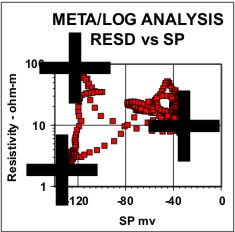Shale Volume from the Spontaneous Potential
The two most common shale indicating logs are the gamma ray (GR) and spontaneous potential (SP) logs. The units of measurement for GR are API units or counts per second, and for SP are millivolts.

The algebraic formula to solve for shale volume from the SP log is a linear interpolation between the minimum and maximum log readings, similar to the GR solution.

1: VSHsp = (SP - SP0) / (SP100 - SP0)
2:  VSHsp = Min(1, Max(0, VSHsp))

Where:
SP = spontaneous potential log reading in zone of interest (mv)
SP0 = spontaneous potential log reading in 100% clean zone (mv)
SP100 = spontaneous potential log reading in shale (mv)
VSHsp = shale volume from spontaneous potential (fractional)COMMENTS:
The SP method is the second most popular approach to shale volume. The SP is reduced by high resistivity, often associated with hydrocarbons or tight zones, so these may appear too shaly by this approach. The method has poor resolution in zones with fresh formation water, or in wells drilled with salty mud. The method works well in radioactive sands, but not in carbonates.

References:
1. The SP Log: Theoretical Analysis and Principles of Interpretation,
H.G. Doll, AIME, 1948.

H.G. Doll, JPT, 1950.Shale Volume from ALPHA Method (OBSOLETE)
In older charts and technical papers, the SP is called the pseudo static SP (PSP) and SP0 is called the static SP (SSP). The same formula was used for shale volume as given above, with SP100 taken as zero. The result is always called ALPHA, the SP reduction factor, and was often equated to shale volume:

3: VSHpsp = ALPHA = PSP / SSP
4:  VSHpsp = Min(1, Max(0, VSHpsp))

This formula is no longer commonly used.RECOMMENDED PARAMETERS:
Range          Default
SP0            -100 to -45         -80 mv
SP100          -10 to +10           0 mv
Choose from crossplot or depth plot

NUMERICAL EXAMPLE:
Assume  SP = -50,  SP0 = -90,  SP100 = 0 mv.

1. Vsh from spontaneous potential log:
VSHsp = (-50 - (-90)) / (0 - (-90)) = 0.44

Page Views ---- Since 01 Jan 2015